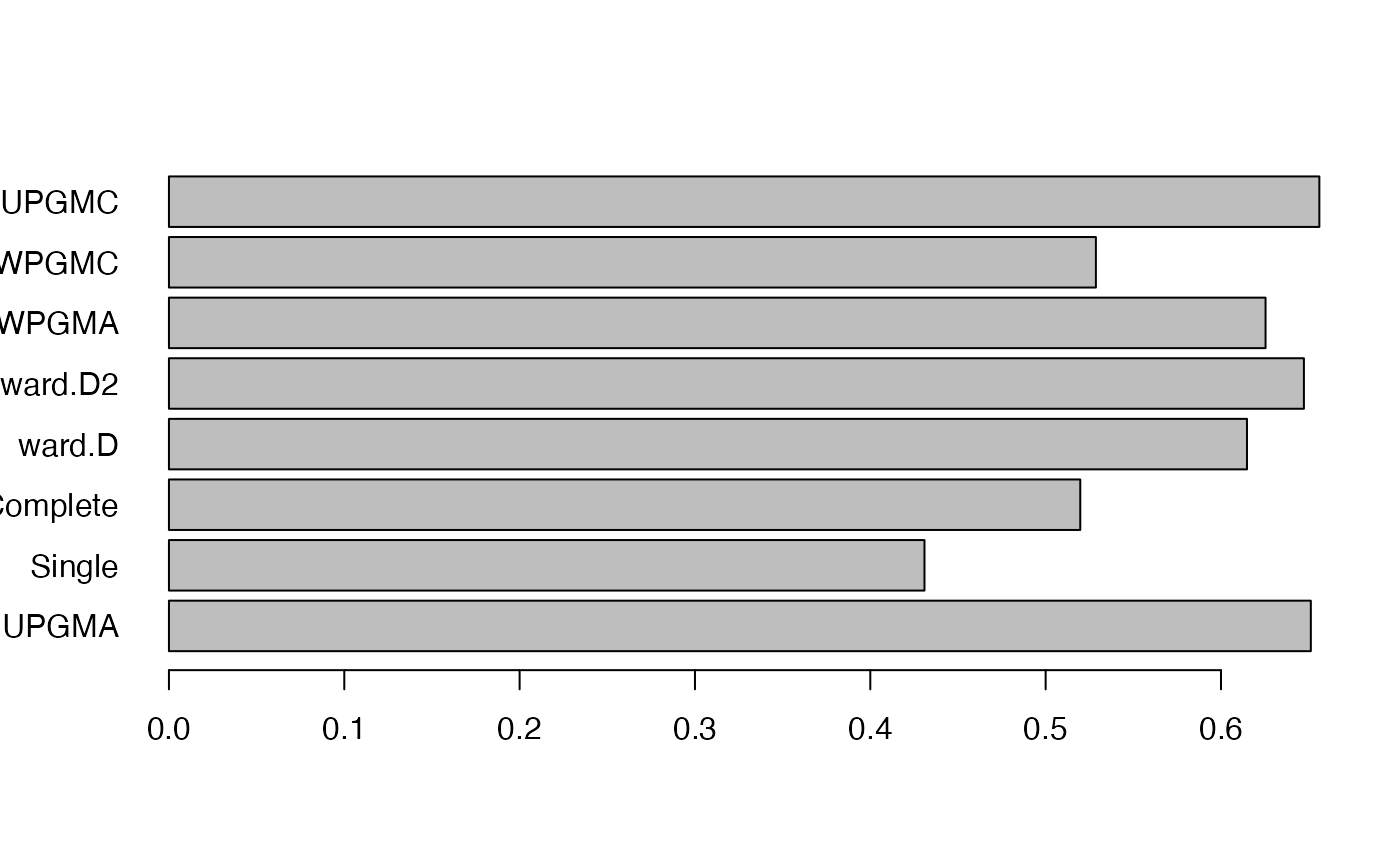This function contrasts different hierarchical clustering algorithms on the phylogenetic beta diversity matrix for degree of data distortion using Sokal & Rohlf’s (1962) cophenetic correlation coefficient.

select_linkage(x)

## Arguments

x

a numeric matrix, data frame or "dist" object.

## Value

• A numeric value corresponding to the good clustering algorithm for the distance matrix

• If plot = TRUE, a barplot of cophenetic correlation for all the clustering algorithms is drawn.

## Examples

data(africa)
tree <- africa$phylo bc <- beta_diss(africa$comm)